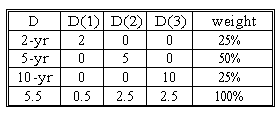### CFA Practice Question

There are 764 practice questions for this topic.

### CFA Practice Question

Consider the following portfolios comprised of 2-year, 5-year and 10-year zero-coupon bonds. D(n) is the key rate duration for the n-year part of the yield curve.If the 2-year key rate shifts up 10 basis points and the 10-year rate shifts down 10 basis points, the value of the portfolio will change by ______.

Change in portfolio's value due to 2-year key rate change: 0.5 x (-10/100) = -0.05%.

Change in portfolio's value due to 10-year key rate change: 2.5 x (10/100) = 0.25%.

The total change in portfolio value is an increase of 0.2%.